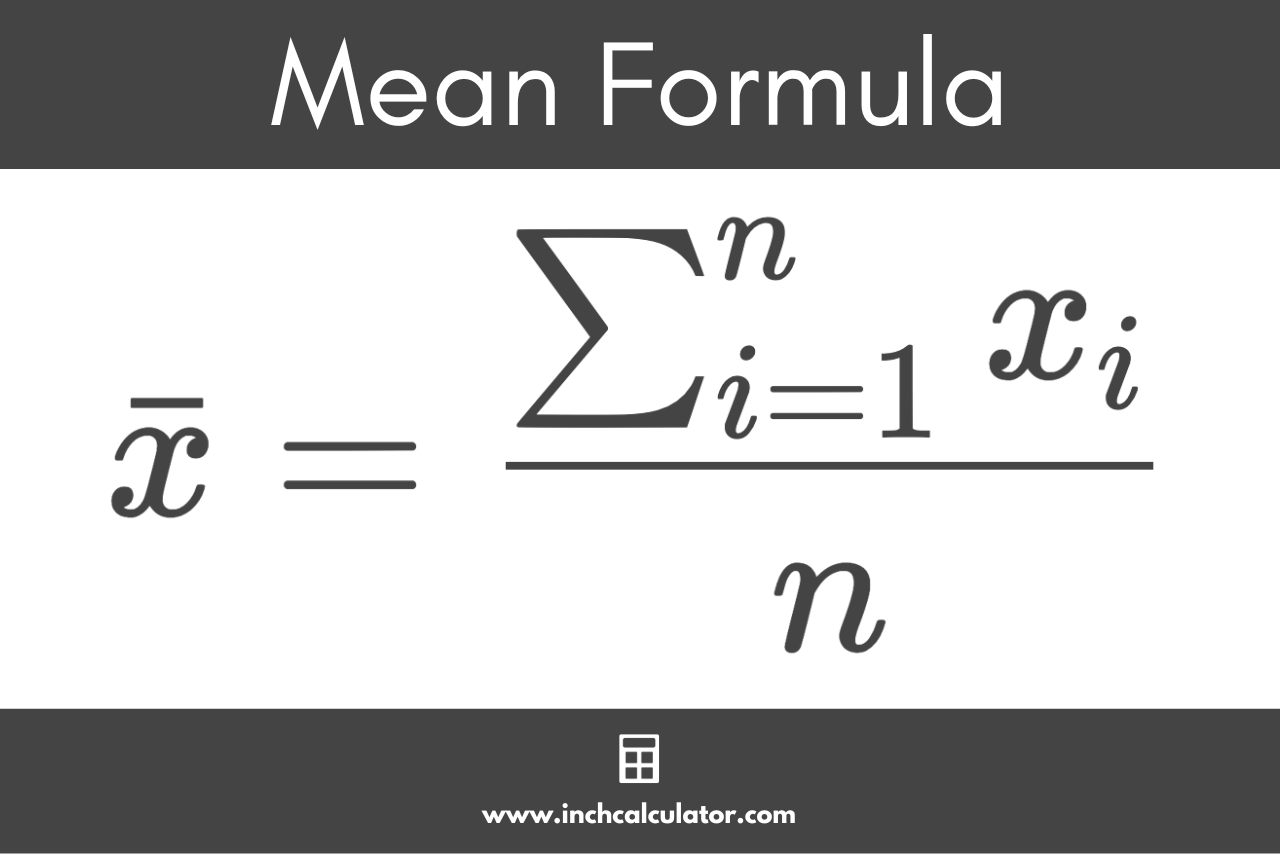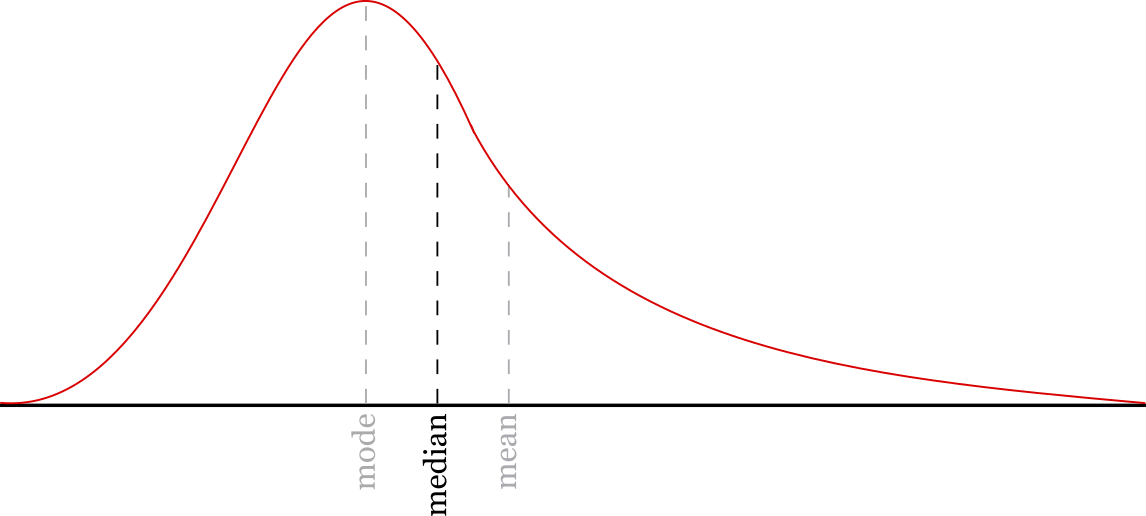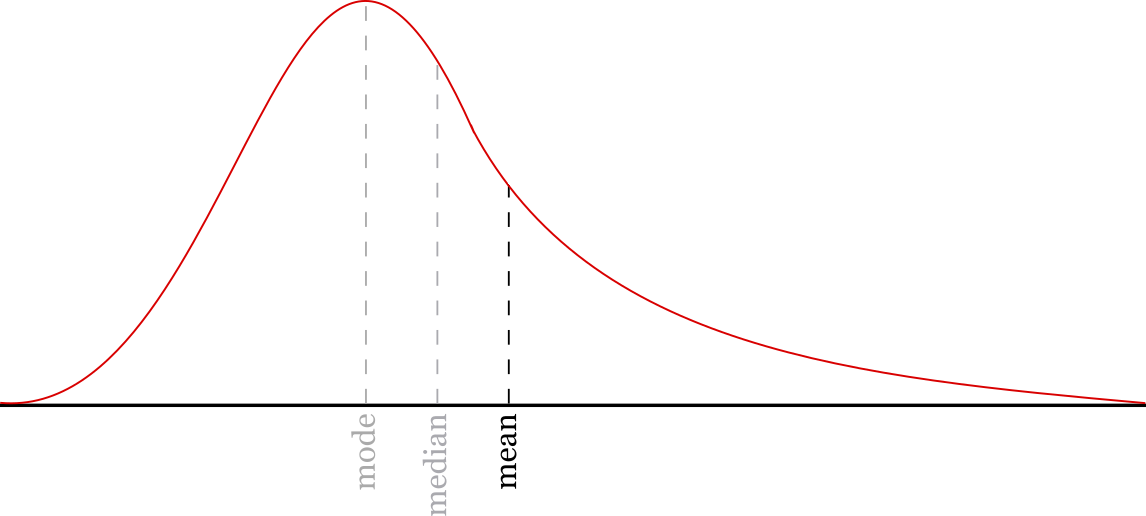# Mean Calculator

Find the mean of a dataset by entering the numbers in the calculator below.

Separate numbers using a comma (,)

## Results:

### Sorted Data:

Learn how we calculated this below

## How to Find the Mean

The mean, or arithmetic mean, is the average value of a set of numbers. Thus, mean and average are the same thing.

The mean and average are one way to express the middle of a collection of numbers. More specifically, the mean is a measure of the central tendency of the group of numbers.

The mean for a sample is denoted (pronounced x-bar), and the population mean is denoted μ (Greek letter mu). It is one of the fundamental observations of data used in summary statistics.

### Mean Formula

You can find the mean using the following formula:

\text{mean}=\bar{x}=\frac{\sum_{i=1}^{n}x_{i}}{n}

Where:
xi = each number in the set
n = total number of values in the set

Thus, the mean is equal to the sum of all the numbers in the dataset divided by the count of the numbers n.

The formula to find the mean is equivalent to the following:

\text{mean}=\bar{x}=\frac{x_{1}+x_{2}+…+x_{n}}{n}

Or put simply:

\text{mean}=\bar{x}=\frac{\text{sum}}{\text{count}}### Steps to Calculate Mean

There are three steps for calculating the mean of a dataset.

1. Step 1: count the total number of values in the set
2. Step 2: add up all the numbers to find the sum
3. Step 3: divide the sum by the count

So, to find the mean, start by finding the number of values in the dataset, then find the sum of all of the values in the set, and finally, divide the sum by the total count of the values.

### Example: Calculating the Mean

Calculate the mean of the following numbers: [3,1,8,12,22,5]

Step 1: count the numbers.
count = 6

Step 2: add up the numbers.
sum = 3 + 1 + 8 + 12 + 22 + 5
sum = 51

Step 3: divide the sum by the count.
x̄ = 51 / 6
x̄ = 8.5

The mean of the numbers above is 8.5.

This is the process for finding the arithmetic mean, but there are several other variations of the mean to measure central tendency.

## Geometric Mean

The geometric mean is another measure of the central tendency of a dataset. Instead of measuring the center using addition and division like the formulas above, it uses multiplication and the root.

### Geometric Mean Formula

The geometric mean can be expressed using the following formula:

\bar{x}=\left ( \prod_{i=1}^{n}x_{i} \right )^{\frac{1}{n}}

This is equivalent to the nth root of the product of the values in the set:

\bar{x} = \sqrt[n]{x_{1} \cdot x_{2} \cdot\cdot\cdot x_{n}}

## Weighted Mean

A weighted mean, sometimes called a weighted average, is an average value that reflects a different amount of importance of each value in a data. It is different from the arithmetic mean since each value contributes to the average more or less, depending on its weight.

### Weighted Mean Formula

The weighted mean can be expressed using the following formula:

\bar{x}=\frac{\sum_{i=1}^{n}\left (x_{i} \cdot w_{i} \right )}{\sum_{i=1}^{n}w_{i}}

The weighted mean is equal to the sum of the products of each weight w and its value x, divided by the sum of the weights w.

This is a simplified representation of the formula:

\bar{x}=\frac{\left (x_{1} \cdot w_{1} \right ) + \left (x_{2} \cdot w_{2} \right ) + … + \left (x_{n} \cdot w_{n} \right )}{w_{1} + w_{2} + … + w_{n}}

## Harmonic Mean

The harmonic mean is yet another measure of central tendency, and it is the reciprocal of the arithmetic mean of the data.

### Harmonic Mean Formula

The harmonic mean can be expressed using the following formula:

\bar{x}=\frac{n}{\sum_{i=1}^{n}\frac{1}{x_{i}}}

The harmonic mean is equal to the count of values in a set of n numbers, divided by the sum of the reciprocals of each value.

This is equivalent to the following simplified formula:

\bar{x}=\frac{n}{\frac{1}{x_{1}}+\frac{1}{x_{2}}+…+\frac{1}{x_{n}}}

## Mean vs. Median

We briefly touched on summary statistics above. Another common observation of numeric data used in summary statistics is the median.

The median is also a measure of the central tendency of numeric data. The median is the middle value or middle number of the dataset rather than the average value.The median represents the mid-point of a number set. Half of the numbers in the set are above the median, and half are below it.

So should you use the mean or the median to describe your data? The mean might be beneficial if the average value of the entire set of data is important.

Since the mean considers each value in the data, it might be a more accurate way to describe the central tendency of the data if the majority of the data are in an evenly distributed range.However, major outliers in the data can skew the mean in one direction. Consider the data: [1,2,3,4,75]

The average value of this data is 17, while the median value is 3.

Since the median only considers the midpoint of the data, it might do a better job of discarding those outliers and reducing the skew. However, there are some cases where those outlier values are important and need to be considered, so the mean might be a better representation of the data in that case.

You might also be interested in our mean, median, & mode calculator.

## References

1. Holmes, A., Illowsky, B., Dean, S., Introductory Business Statistics - 2.4 Sigma Notation and Calculating the Arithmetic Mean, https://openstax.org/books/introductory-business-statistics/pages/2-4-sigma-notation-and-calculating-the-arithmetic-mean